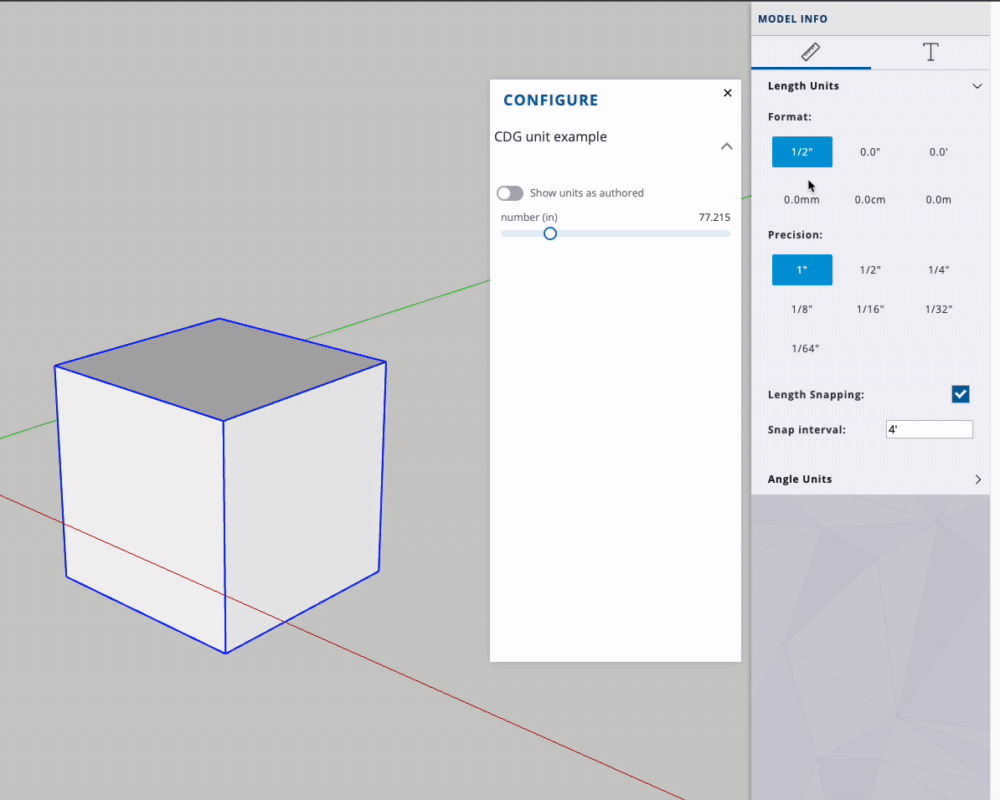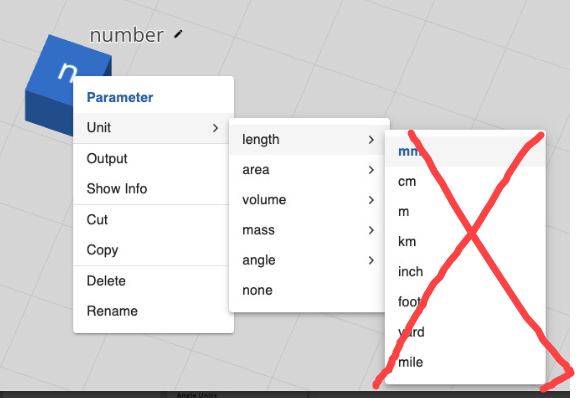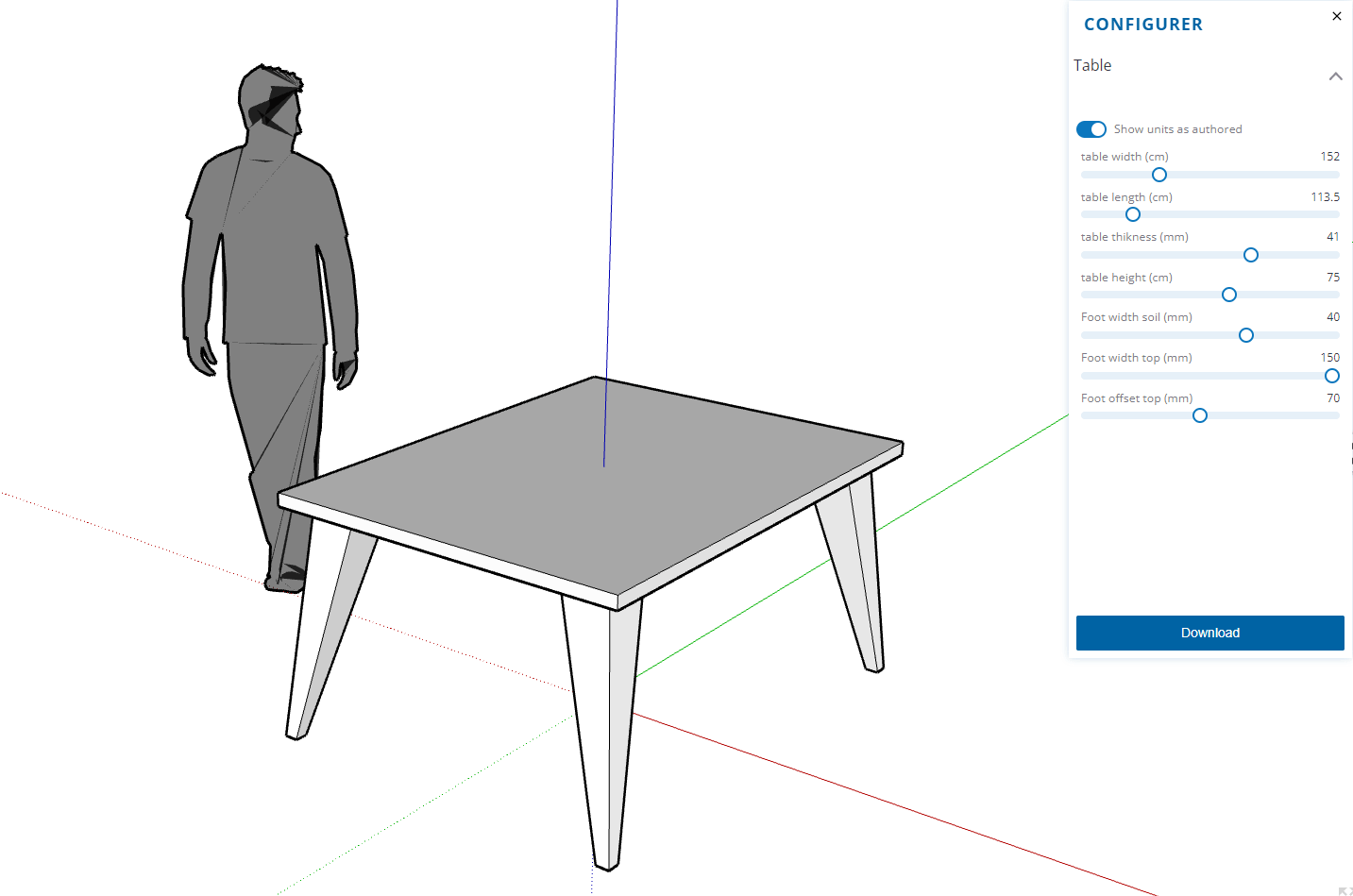# Units of input parameters are not converted to units of the graph

Hello
I did a small test of simple addition of two numbers A is set with the unit centimeters and B in millimeters.
The graph is set in meters.
The sum does not consider the units and the result of the addition is a basic sum of the two numbers and not the sum of the values converted into the unit of the graph.

I think it’s important to fix this.

Hi Sim!

The graph is actually unitless. A long story short, for the graph to have units, values would need to be aware of their unit (which is not the case and is far more complicated than it sounds to implement).

A long story of middling length…

We support display units for parameters, and the graph output length unit (which is really the “size” of the model when displayed in 3d).

For display units: A ‘width’ parameter, with a display unit mm and a value of 15 doesn’t output 15mm, it simply outputs 15. The display unit can however be used to translate how 15 is displayed in things like the configuration view (seen in SketchUp). This means that if the user in SketchUp sets their working unit to feet, the configuration view can be adjusted to display 15mm as if it were 0.0492126ft).

For output units: A graph that builds a house has no unit. The nodes of the graph might create a house that is [10.5,16,5] in XYZ dimensions though. While this model has no unit in the graph the output length unit can be set to ‘m’. This means that when the 3dview (web3d in Creator, or SketchUps own viewer in SketchUp) creates the model in 3d space, it can translate to its own display space (metres in web3d, inches in SketchUp). This space is actually different to the ‘working’ unit you find in SketchUp, SketchUp is fundamentally an inch modeler, even if the working unit set by the user is set to metres.

Good evening Keith
I don’t really understand the reasoning to propose different input units for the input parameters and one unit for the graph if there is no consistency between them.
Suggesting to the user to enter with the units of his SketchUp model is interesting, but his values ​​must be converted into the unit of the graph. there is thus consistency.
I take for example:
An input parameter set to 10 mm, with a graph set to millimeters as well.
An expression node if a>=4? 1:0

Assuming the user is working in inches on their Sketchup model, and turn off graph units.

A formula that makes a comparison: if value greater than 4 (implying 4 mm, because the graph is in millimeters.) Then 1 , otherwise 0.
4mm is different from 4 inches.

I do not agree ! The graph must have a unit! Whether the user enters with the units provided by the graph or that of his model. Inputs must be converted into graph units and then interpreted by the graph.

I hope to convince you of the imperious necessity of consistency in the unity of the graph. And its entries.

I think we have perhaps miscommunicated to the user what setting a unit on a parameter actually does in the graph, and how this relationship works with what is displayed to the user in SketchUp.

For example:

This makes it look like this number has a unit of ‘mm’, and will flow through the graph acting as if it is a length. This unfortunately isn’t the case.

What that unit is actually for is how that number is multiplied and displayed within the configuration context in SketchUp (or any other viewer):Perhaps we should call this a ‘configuration display unit’ or ‘display unit’ or ‘configuration unit’ or ‘intended unit’. Would one of those be a better description of the above behavior?

@keith_thurlow This is indeed inconsistent. How about you remove the mm, cm,… from the number node and just let the user classify whether it’s a length unit etc. Since the actual unit is already set in the overall graph, you can then add the respective unit to a number parameter when it’s shown to the user in the parameter dialog. I.e. if the graph unit is set to inches, then a length number just gets the inch label added.

Alternatively, you can have the LC query the unit from the current SketchUp model into which the LC has been inserted and then convert the parameter sliders on the fly, complete with unit label display.Good evening
Where I stumble on your respective reasoning is that in 90% of cases, we seek to model real objects that have a size that can be measured in inches or mm, it doesn’t matter, the physical size is always the same and must be the same according to a defined conversion rate. A table at a height of 75 cm, a seat of 45 cm.
If the graph designer defines the unit of the graph in inches and proposes input parameters in mm, the nodes must return output values ​​in inches. Like SketchUp does, which converts all lengths to inches. If the end user prefers inches and turns on the inch model units option, no problem, the nodes take inches and return their inches (pun intended).
Consistency of units is essential! And taking the example of the 75 cm high table, you may prefer to use millimeters for certain parameters to ask for the thickness of the top, the width of the legs.

You need to review the number and integer nodes so that the conversions are carried out according to the unit defined on the node towards the unit of the graph.

@alexschreyer SketchUp does currently change the unit displayed in the configure panel, based on the user’s model unit (Web does this dynamically, desktop requires that the user closes/opens the configure panel on model unit change). Does the current workflow meet the alternative proposal @alexschreyer ?

@simjoubert if we go back to the original image we can see the graph is correctly adding two unitless values. The issue is that we have set expectations that differ from your own, and have poorly communicated them.
To correct the image, the fix is simply to divide the ‘cm’ value before adding if you want the ‘add’ to be correct relative to the output space.

Units are a tricky thing, and we need to be better at setting users’ expectations on unit flows in Creator. As you can see from this thread, it is a difficult topic (or perhaps I’m just struggling to communicate the difficulties clearly).

Parametric modelers are often unitless, as units often hurt more than help graphs with complex parametric relationships. Grasshopper and Houdini are both unitless (but they output models into a space that has units like we do).

If you are adding two lengths, it sounds useful for the ‘add’ node to be unit aware. As we start mixing things like count + length + volume in the ‘add’ we are in trouble. Should multiplying two lengths give a unit that is an area? Probably not because that assumes that the lengths are perpendicular to each other.
Those are simple problems introduced within a node itself, you would also have to consider how data flows between nodes. How should a length value interact with an input that is supposed to set a scale instead of size?

When you see other applications show units, it is likely that some parsing magic is occurring on the input or output field, but not within the parametric relationships of the model itself.

I think I don’t mind the graph unitless, however, to make it easier to work with a unitless graph, i think it would be helpful to have a unit conversion node. One where you can set the input and output units and just run data through it, and it automatically converts the number for you. I know this can be done with a multiply or division node but a conversion node would make the graph look cleaner and easier to understand. That, and communicating the intent of the parameter units. I think “Display Unit” makes sense.

1 Like

Changing units dynamically makes sense and is working well in SketchUp. I am still wondering whether setting the actual unit (in, mm,…) on a number input makes sense in the unitless environment of a graph. If we only specified whether it’s a length or just a number, then the overall graph unit can be displayed to the user automatically. I.e. eliminating the third menu level (as shown in my image). I guess a use case is when there are parameters that need to be entered in inches and others in feet (or similar). But then it’s left to the graph programmer/creator to make sure the math is appropriate. I somehow prefer the rigid “single unit for the entire graph” approach, however.

2 Likes

Hello
I realized a small lC Table with voluntarily subunits of the meter for certain parameter followed by a multiplication of conversion.
In SU Online and SU Pro, the deactivation of the units provided by that of the model takes into account the converted max and min bounds.

So all is well in the best of worlds.
So my position is:
Keeping the possibility of a different unit than that of the graph is a good thing in order to be able to meet business requirements and certain customs. A TV screen the diagonal is expressed in inches even if I am in France!I also think a convert node could be handy to convert to graph unit.
Or provide a Boolean to convert to the graph unit if the graph units are kept by the end user. We would thus gain a node, conversion or multiplication.Graph Table

1 Like

Yeah, a node like that could be something to look at. We have to be careful not to reinforce the expectation that the graph has units of values when it is really just a preset set of divisions/multiplications for values.

Ah, just to be certain, would that mean the user can “request” a specific unit per parameter rather than relying on the model unit to set all parameters during configuration?

Hello Keith
3 possibilities :

• The number node have a boolean option to convert the exit value in the graph unite.
• The number node have 2 exits, the first is the value, the 2nd is the convert value in graph unit
• You create a new node conversion, it will have two choice lists options : unit entrance, and unit exit.

For the two first possibilities, the value is only converted if the units of graph parameters are not used by the users